﻿ 基于PCA的人脸识别算法在课堂考勤中的应用研究 PCA-Based Face Recognition Algorithm in Class Attendance

Computer Science and Application
Vol.08 No.03(2018), Article ID:24235,12 pages
10.12677/CSA.2018.83041

PCA-Based Face Recognition Algorithm in Class Attendance

Meixiang Wu, Yuanyuan Deng, Fenghua Pei, Songsen Yu

School of Software, South China Normal University, Nanhai Guangdong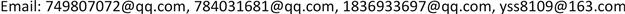Received: Mar. 9th, 2018; accepted: Mar. 21st, 2018; published: Mar. 28th, 2018ABSTRACT

This paper mainly studies the PCA-based face recognition algorithm. It firstly preprocesses the images collected, detects face based on AdaBoost classification algorithm, and then uses PCA algorithm for face recognition and matching. All of above can be applied to mobile attendance system. This system combines face recognition technology and class attendance, to realize real-time attendance and statistics on android client. After experimental testing, system reaches an accuracy of over 75%.1. 引言

2. 现有研究成果对比分析

3. 人脸检测

3.1. Haar分类器算法

Opencv自带了haar分类器，Haar分类器算法的要点如下：

· 使用Haar-like特征做检测。

· 使用积分图(Integral Image)对Haar-like特征求值进行加速。

· 使用筛选式级联把强分类器级联到一起，提高准确率。

1) 给定训练样本集S，共N个样本，其中X和Y分别对应于正样本和负样本；T为训练的最大循环次数；

2) 初始化样本权重为1/N，即为训练样本的初始概率分布；

3) 第一次迭代训练N个样本，得到第一个最优弱分类器;

4) 提高上一次迭代中被误判的样本的权重；

5) 将新的样本和上次分错的样本放在一起进行新一轮的训练;

6) 循环执行4~5步骤，T轮后得到T个最优弱分类器。

7) 将T个最优弱分类器组合而得强分类器，组合方式(1)所示：

$C\left(x\right)=\left\{\begin{array}{l}1\text{}{\sum }_{t=1}^{T}{\alpha }_{t}{h}_{t}\left(x\right)\ge \frac{1}{2}{\sum }_{t}^{T}{\alpha }_{t}\\ 0\text{}其他\end{array}$ (1)

${C}_{\text{FINAL}}\left(x\right)=\mathrm{sin}\left(\sum _{i=1}^{M}{a}_{i}{C}_{i}\left(x\right)\right)$ (2)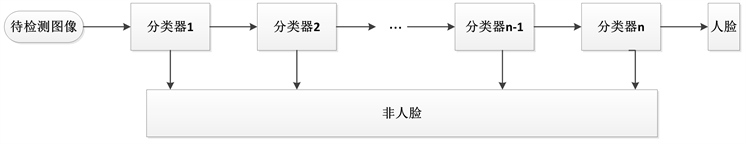Figure 1. Face detection classifier training method

4. 人脸图像预处理

4.1. 图像去噪

SNN均值滤波算法

4.2. 图像增强

1) 求出原图 ${\left[f\left(x,y\right)\right]}_{M×N}$ 的灰度直方图，用256维的向量h表示。则

$h\left(x\right)=p\left(x\right)=\frac{{n}_{k}}{N}\text{\hspace{0.17em}}\text{\hspace{0.17em}}\left(k=0,1,\cdots ,L-1\right)$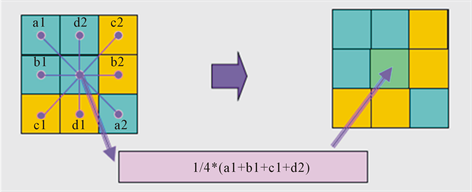Figure 2. SNN Algorithm schematicFigure 3. KNN mean filtering algorithm denoising effect chart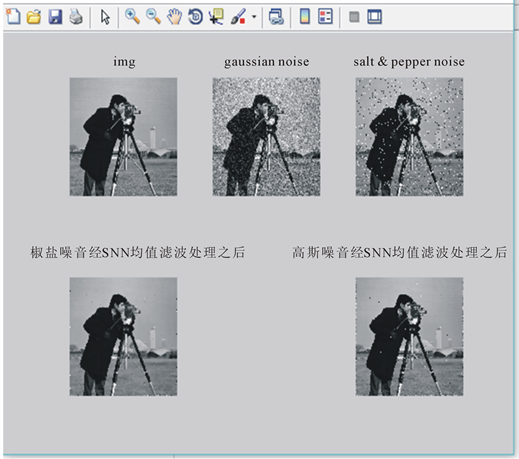Figure 4. SNN mean filtering algorithm denoising effect chart

2) 由直方图均衡化映射函数进行直方图的均衡化。则映射函数为：

$g\left(x,y\right)=\left\{\begin{array}{l}\left(L-1\right),\text{\hspace{0.17em}}\text{\hspace{0.17em}}\text{\hspace{0.17em}}\sum _{k=0}^{f\left(x,y\right)}p\left(x\right)f\left(x,y\right)\ne 0\\ 0,\text{\hspace{0.17em}}\text{\hspace{0.17em}}\text{\hspace{0.17em}}\text{\hspace{0.17em}}\text{\hspace{0.17em}}\text{\hspace{0.17em}}\text{\hspace{0.17em}}\text{\hspace{0.17em}}\text{\hspace{0.17em}}\text{\hspace{0.17em}}\text{\hspace{0.17em}}\text{\hspace{0.17em}}f\left(x,y\right)=0\end{array}$

4.3. 图像分割

1) 对待分割的图像 ${\left[f\left(x,y\right)\right]}_{m×n}$ 按照某锐化算子(如sobel算子)求梯度图 $\nabla {\left[f\left(x,y\right)\right]}_{m×n}$

2) 按照如下公式求梯度图 $\nabla {\left[f\left(x,y\right)\right]}_{M×N}$ 的加权灰度直方图 $h\left(k\right)\left(k=0,1,2,\cdots ,255\right)$ ，即Figure 5. Original imageFigure 6. Histogram equalization effect chart

$h\left(k\right)=w\left(k\right)\cdot N\left(k\right),\text{\hspace{0.17em}}\text{\hspace{0.17em}}k=0,1,2,\cdots ,255$

3) 将加权梯度直方图，带入最大熵阈值方法，求出 $\nabla {\left[f\left(x,y\right)\right]}_{m×n}$ 的分割阈值。

5. 人脸识别

1) 将X的每一行(代表一个属性字段)进行零均值化，即减去这一行的均值，使每一维的均值为0。即

$X\left[n,m\right]=X\left[n,m\right]-\frac{1}{m}\sum _{1}^{m}X\left[n,m\right]$ (1)

2) 求出协方差：数学上可以用两个字段的协方差表示其相关性，由于已经让每个字段均值为0，则：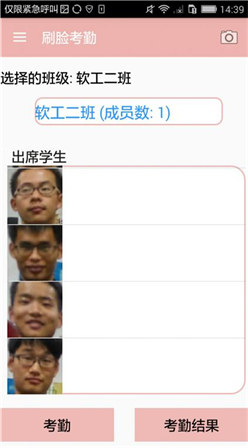Figure 7. Image segmentation effect chart

$Cov\left(a,b\right)=\frac{1}{m}\sum _{i=1}^{m}{a}_{i}{b}_{i}$ (2)

3) 协方差矩阵：

$C=\frac{1}{m}\sum _{i=1}^{m}\left({X}_{i}-\overline{X}\right){\left({X}_{i}-\overline{X}\right)}^{\text{T}}=\frac{1}{m}X\cdot {X}^{\text{T}}$ (3)

4) 求出协方差矩阵的特征值及对应的特征向量。

5) 将特征向量按对应特征值大小从上到下按行排列成矩阵，取前k行组成矩阵P。

6) Y = PXY = PX即为降维到k维后的数据。

6. 移动端设计与实现

6.1. 系统设计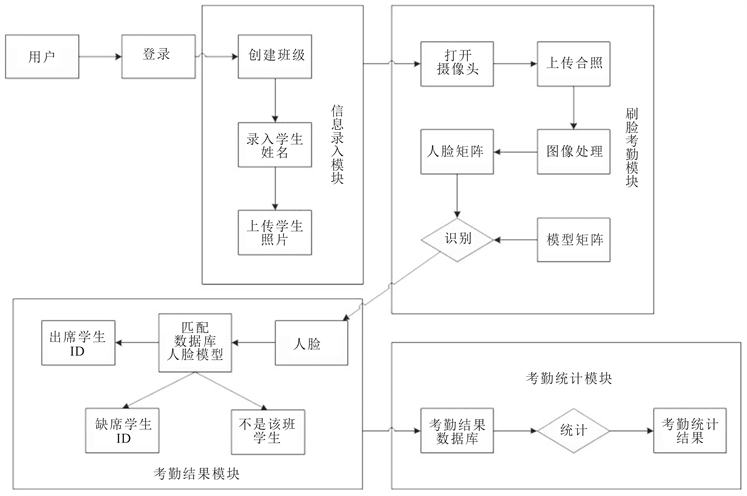Figure 8. System flow chart

6.2. 系统主要功能

6.3. 技术选型

6.3.1. 服务端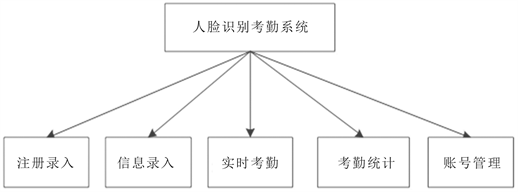Figure 9. System function chart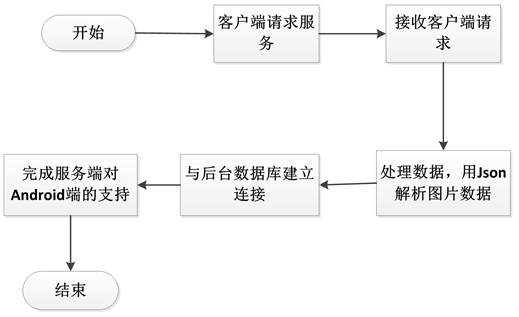Figure 10. Service main flow chart

6.3.2. 数据库

6.4. 效果展示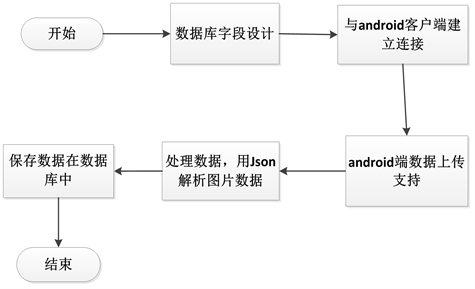Figure 11. Database main flow chart(a)(b)(c)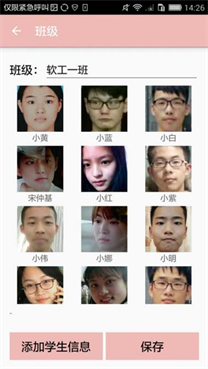(d)

Figure 12. Information entry effect display. (a): Create class name; (b): Create student name; (c): Upload student photo; (d): The total number of student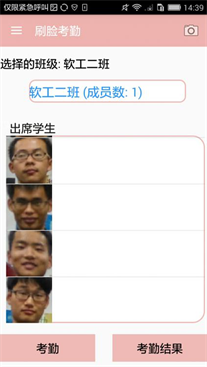(a)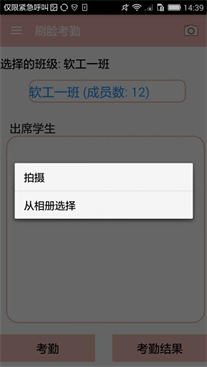(b)(c)

Figure 13. Brush face attendence effect display. (a): Select attendance class; (b): Upload student photo; (c): Click attendence button

7. 结语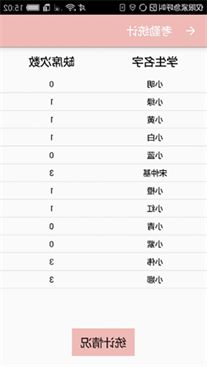(a)(b)(c)

Figure 14. Attendance results and attendance statistics. (a): First attendence result; (b): Second attendence result; (c): Comprehensive statistics

PCA-Based Face Recognition Algorithm in Class Attendance[J]. 计算机科学与应用, 2018, 08(03): 366-377. https://doi.org/10.12677/CSA.2018.83041

1. 1. 赵荣刚, 贺庆民. 计算机人脸识别技术的应用[J/OL]. 电子技术与软件工程, 2018(4): 137.

2. 2. 孔安. 基于PCA和LDA算法的人脸识别考勤管理系统的设计与实现[D]: [硕士学位论文]. 长沙: 湖南大学, 2016.

3. 3. 马园园. 人脸识别技术与考勤系统应用研究[D]: [硕士学位论文]. 南京: 南京邮电大学, 2017.

4. 4. 郝雪夷, 张忠清. 基于手机定位考勤系统的设计与实现[J]. 电子世界, 2017(13): 135-136.

5. 5. 王飞. 基于深度学习的人脸识别算法研究[D]: [硕士学位论文]. 兰州: 兰州交通大学, 2017.

6. 6. 周松锋, 戴曙光. 改进的PCA人脸识别算法研究[J/OL]. 软件导刊, 2018: 1-4.

7. 7. 袁程波. 基于PCA和SVM的人脸识别关键技术研究与实现[D]: [硕士学位论文]. 四川: 电子科技大学, 2017.

8. 8. 李玮. 智慧课堂管理系统中人脸识别考勤技术的研究与实现[D]: [硕士学位论文]. 武汉: 华中师范大学, 2017.

9. 9. 单存杰. 教室场景中学生定位与身份识别[D]: [硕士学位论文]. 武汉: 华中师范大学, 2016.